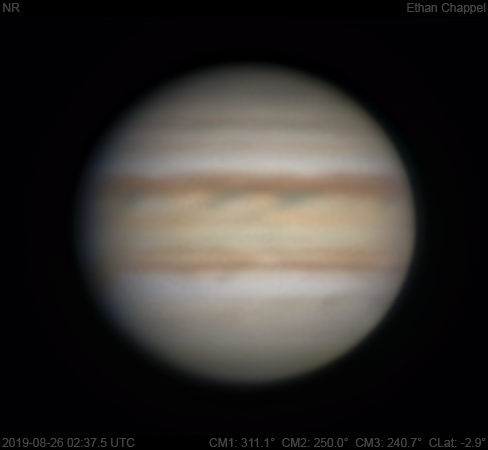# Jupiter 2019-08-26 02:37 UTC

CM1: 311.10°

CM2: 250.00°

CM3: 240.70°

CLat: -2.90°

Description

My first time since late-April imaging next to a parking lot at my university. Conditions were bad for imaging and the optical tube was warm, causing intense heat plumes that worsened the situation. The gusts of wind were intense enough to push my laptop off the table if I didn't hold it down.

Notes:

• White Oval Z, visible as a notch in the North Equatorial Belt, is rising.
• The Equatorial Zone is orange.
• The Great Red Spot is rising.
• An SEBs ring is approaching the central meridian.

Equipment

Celestron C8 EdgeHD

ZWO ASI174MM

Celestron X-Cel 3x

Logs
```FireCapture v2.6  Settings
------------------------------------
Observer=Ethan Chappel
Camera=ZWO ASI174MM
Filter=B
Profile=Jupiter
Diameter=39.65"
Magnitude=-2.26
CMI=315.0° CMII=253.8° CMIII=244.5°  (during mid of capture)
FocalLength=6500mm (F/23)
Resolution=0.19"
Filename=2019-08-26-0243_1-EC-B-Jup.ser
Date=2019_08_26
Start=02_42_08.620
Mid=02_43_08.623
End=02_44_08.627
Start(UT)=02_42_08.620
Mid(UT)=02_43_08.623
End(UT)=02_44_08.627
Duration=120.007s
Date_format=yyyy_MM_dd
Time_format=HH_mm_ss
LT=UT -6h
Frames captured=7977
File type=SER
Binning=no
Bit depth=8bit
Debayer=no
ROI=480x284
ROI(Offset)=8x8
FPS (avg.)=66
Shutter=15.00ms
Gain=400 (100%)
HighSpeed=off
SoftwareGain=10 (off)
AutoExposure=off
AutoGain=off
AutoHisto=75 (off)
USBTraffic=80 (off)
Gamma=50 (off)
FPS=100 (off)
Brightness=1 (off)
Histogramm(min)=0
Histogramm(max)=123
Histogramm=48%
Noise(avg.deviation)=2.76
AutoAlign=false
PreFilter=none
Limit=120 Seconds
Sensor temperature=43.1°C
FireCapture v2.6  Settings
------------------------------------
Observer=Ethan Chappel
Camera=ZWO ASI174MM
Filter=G
Profile=Jupiter
Diameter=39.65"
Magnitude=-2.26
CMI=310.7° CMII=249.6° CMIII=240.3°  (during mid of capture)
FocalLength=6500mm (F/23)
Resolution=0.19"
Filename=2019-08-26-0236_1-EC-G-Jup.ser
Date=2019_08_26
Start=02_35_10.918
Mid=02_36_10.918
End=02_37_10.918
Start(UT)=02_35_10.918
Mid(UT)=02_36_10.918
End(UT)=02_37_10.918
Duration=120.000s
Date_format=yyyy_MM_dd
Time_format=HH_mm_ss
LT=UT -6h
Frames captured=11949
File type=SER
Binning=no
Bit depth=8bit
Debayer=no
ROI=480x284
ROI(Offset)=8x8
FPS (avg.)=99
Shutter=10.00ms
Gain=370 (92%)
HighSpeed=off
SoftwareGain=10 (off)
AutoExposure=off
AutoGain=off
AutoHisto=75 (off)
USBTraffic=80 (off)
Gamma=50 (off)
FPS=100 (off)
Brightness=1 (off)
Histogramm(min)=0
Histogramm(max)=129
Histogramm=50%
Noise(avg.deviation)=1.98
AutoAlign=false
PreFilter=none
Limit=120 Seconds
Sensor temperature=43.8°C
FireCapture v2.6  Settings
------------------------------------
Observer=Ethan Chappel
Camera=ZWO ASI174MM
Filter=R
Profile=Jupiter
Diameter=39.65"
Magnitude=-2.26
CMI=309.0° CMII=247.9° CMIII=238.6°  (during mid of capture)
FocalLength=6500mm (F/23)
Resolution=0.19"
Filename=2019-08-26-0233_3-EC-R-Jup.ser
Date=2019_08_26
Start=02_32_21.457
Mid=02_33_21.457
End=02_34_21.458
Start(UT)=02_32_21.457
Mid(UT)=02_33_21.457
End(UT)=02_34_21.458
Duration=120.001s
Date_format=yyyy_MM_dd
Time_format=HH_mm_ss
LT=UT -6h
Frames captured=7976
File type=SER
Binning=no
Bit depth=8bit
Debayer=no
ROI=480x284
ROI(Offset)=8x8
FPS (avg.)=66
Shutter=15.00ms
Gain=370 (92%)
HighSpeed=off
SoftwareGain=10 (off)
AutoExposure=off
AutoGain=off
AutoHisto=75 (off)
USBTraffic=80 (off)
Gamma=50 (off)
FPS=100 (off)
Brightness=1 (off)
Histogramm(min)=0
Histogramm(max)=127
Histogramm=49%
Noise(avg.deviation)=1.96
AutoAlign=false
PreFilter=none
Limit=120 Seconds
Sensor temperature=43.8°C
```